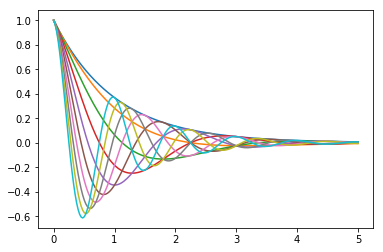# Matplotlib¶

## Overview¶

We’ve already generated quite a few figures in these lectures using Matplotlib.

Matplotlib is an outstanding graphics library, designed for scientific computing, with

• high-quality 2D and 3D plots
• output in all the usual formats (PDF, PNG, etc.)
• LaTeX integration
• fine-grained control over all aspects of presentation
• animation, etc.

### Matplotlib’s Split Personality¶

Matplotlib is unusual in that it offers two different interfaces to plotting.

One is a simple MATLAB-style API (Application Programming Interface) that was written to help MATLAB refugees find a ready home.

The other is a more “Pythonic” object-oriented API.

For reasons described below, we recommend that you use the second API.

But first, let’s discuss the difference.

## The APIs¶

### The MATLAB-style API¶

Here’s the kind of easy example you might find in introductory treatments

In [ ]:
%matplotlib inline
import matplotlib.pyplot as plt
plt.rcParams["figure.figsize"] = (10, 6) #set default figure size
import numpy as np

x = np.linspace(0, 10, 200)
y = np.sin(x)

plt.plot(x, y, 'b-', linewidth=2)
plt.show()


This is simple and convenient, but also somewhat limited and un-Pythonic.

For example, in the function calls, a lot of objects get created and passed around without making themselves known to the programmer.

Python programmers tend to prefer a more explicit style of programming (run import this in a code block and look at the second line).

This leads us to the alternative, object-oriented Matplotlib API.

### The Object-Oriented API¶

Here’s the code corresponding to the preceding figure using the object-oriented API

In [ ]:
fig, ax = plt.subplots()
ax.plot(x, y, 'b-', linewidth=2)
plt.show()


Here the call fig, ax = plt.subplots() returns a pair, where

• fig is a Figure instance—like a blank canvas.
• ax is an AxesSubplot instance—think of a frame for plotting in.

The plot() function is actually a method of ax.

While there’s a bit more typing, the more explicit use of objects gives us better control.

This will become more clear as we go along.

### Tweaks¶

Here we’ve changed the line to red and added a legend

In [ ]:
fig, ax = plt.subplots()
ax.plot(x, y, 'r-', linewidth=2, label='sine function', alpha=0.6)
ax.legend()
plt.show()


We’ve also used alpha to make the line slightly transparent—which makes it look smoother.

The location of the legend can be changed by replacing ax.legend() with ax.legend(loc='upper center').

In [ ]:
fig, ax = plt.subplots()
ax.plot(x, y, 'r-', linewidth=2, label='sine function', alpha=0.6)
ax.legend(loc='upper center')
plt.show()


If everything is properly configured, then adding LaTeX is trivial

In [ ]:
fig, ax = plt.subplots()
ax.plot(x, y, 'r-', linewidth=2, label='$y=\sin(x)$', alpha=0.6)
ax.legend(loc='upper center')
plt.show()


Controlling the ticks, adding titles and so on is also straightforward

In [ ]:
fig, ax = plt.subplots()
ax.plot(x, y, 'r-', linewidth=2, label='$y=\sin(x)$', alpha=0.6)
ax.legend(loc='upper center')
ax.set_yticks([-1, 0, 1])
ax.set_title('Test plot')
plt.show()


## More Features¶

Matplotlib has a huge array of functions and features, which you can discover over time as you have need for them.

We mention just a few.

### Multiple Plots on One Axis¶

It’s straightforward to generate multiple plots on the same axes.

Here’s an example that randomly generates three normal densities and adds a label with their mean

In [ ]:
from scipy.stats import norm
from random import uniform

fig, ax = plt.subplots()
x = np.linspace(-4, 4, 150)
for i in range(3):
m, s = uniform(-1, 1), uniform(1, 2)
y = norm.pdf(x, loc=m, scale=s)
current_label = f'$\mu = {m:.2}$'
ax.plot(x, y, linewidth=2, alpha=0.6, label=current_label)
ax.legend()
plt.show()


### Multiple Subplots¶

Sometimes we want multiple subplots in one figure.

Here’s an example that generates 6 histograms

In [ ]:
num_rows, num_cols = 3, 2
fig, axes = plt.subplots(num_rows, num_cols, figsize=(10, 12))
for i in range(num_rows):
for j in range(num_cols):
m, s = uniform(-1, 1), uniform(1, 2)
x = norm.rvs(loc=m, scale=s, size=100)
axes[i, j].hist(x, alpha=0.6, bins=20)
t = f'$\mu = {m:.2}, \quad \sigma = {s:.2}$'
axes[i, j].set(title=t, xticks=[-4, 0, 4], yticks=[])
plt.show()


### 3D Plots¶

Matplotlib does a nice job of 3D plots — here is one example

In [ ]:
from mpl_toolkits.mplot3d.axes3d import Axes3D
from matplotlib import cm

def f(x, y):
return np.cos(x**2 + y**2) / (1 + x**2 + y**2)

xgrid = np.linspace(-3, 3, 50)
ygrid = xgrid
x, y = np.meshgrid(xgrid, ygrid)

fig = plt.figure(figsize=(10, 6))
ax.plot_surface(x,
y,
f(x, y),
rstride=2, cstride=2,
cmap=cm.jet,
alpha=0.7,
linewidth=0.25)
ax.set_zlim(-0.5, 1.0)
plt.show()


### A Customizing Function¶

Perhaps you will find a set of customizations that you regularly use.

Suppose we usually prefer our axes to go through the origin, and to have a grid.

Here’s a nice example from Matthew Doty of how the object-oriented API can be used to build a custom subplots function that implements these changes.

Read carefully through the code and see if you can follow what’s going on

In [ ]:
def subplots():
"Custom subplots with axes through the origin"
fig, ax = plt.subplots()

# Set the axes through the origin
for spine in ['left', 'bottom']:
ax.spines[spine].set_position('zero')
for spine in ['right', 'top']:
ax.spines[spine].set_color('none')

ax.grid()
return fig, ax

fig, ax = subplots()  # Call the local version, not plt.subplots()
x = np.linspace(-2, 10, 200)
y = np.sin(x)
ax.plot(x, y, 'r-', linewidth=2, label='sine function', alpha=0.6)
ax.legend(loc='lower right')
plt.show()


The custom subplots function

1. calls the standard plt.subplots function internally to generate the fig, ax pair,
2. makes the desired customizations to ax, and
3. passes the fig, ax pair back to the calling code.

## Exercises¶

### Exercise 1¶

Plot the function

$$f(x) = \cos(\pi \theta x) \exp(-x)$$

over the interval $[0, 5]$ for each $\theta$ in np.linspace(0, 2, 10).

Place all the curves in the same figure.

The output should look like this## Solutions¶

### Exercise 1¶

Here’s one solution

In [ ]:
def f(x, θ):
return np.cos(np.pi * θ * x ) * np.exp(- x)

θ_vals = np.linspace(0, 2, 10)
x = np.linspace(0, 5, 200)
fig, ax = plt.subplots()

for θ in θ_vals:
ax.plot(x, f(x, θ))

plt.show()